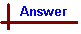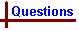Real Gas Problems (Advanced) Shortcut to Questions Q: 1 2 3 4 5 6 1 Carbon dioxide gas (1.00 mole) at 373 K occupies 536 mL at 50.0 atmosphere pressure. What is the calculated value of the pressure using (i) Ideal gas equation (ii) Van der Waals equation? [Data - Van der Waals constants for carbon dioxide: a = 3.61 L2 atm mol-2; b = 0.0428 L mol-1] Calculate the % deviation of each value from that observed.2 Predict which of the substances, NH3, N2, CH2Cl2, Cl2, CCl4 has (i) the smallest van der Waals "a" constant (ii) the largest "b" constant.3 (i) Using Van der Waals equation, calculate the temperature of 20.0 mole of helium in a 10.0 litre cylinder at 120 atmosphere pressure. [Data - Van der Waals constants for helium: a = 0.0341 L2 at mol-2; b = 0.0237 L mol-1] (ii) Compare this value with the temperature calculated from the ideal gas equation.4 A particular reaction in the gas phase has an activation energy of 8.000 kJ mol-1. For 1.00 mole of gas, calculate the number of molecules which exceed this activation energy at (a) 300 K (b) 400 K5 At 250 K, the activation energy for a gas phase reaction was determined to be 6.500 kJ mol-1. What percentage of gaseous molecules would be expected to have less than this energy at 250 K?6 For 1.00 mole of a particular gas, the average molecular energy is found to be 1.300 kJ mol-1 at 298 K. Approximately how many molecules have at least five times the average molecular kinetic energy.Real Gas Problems (Answers) Preamble: Assumptions underlying the concept of an ideal gas are: (i) collisions involving gas phase molecules are perfectly elastic (ii) the volume of the molecules is insignificant compared with the volume of their container. However, for real gases attractive forces exist between molecules when they collide, giving rise to "sticky collisions", so that at any instant there are actually fewer separate particles present than the number of individual molecules that the ideal gas model assumes. Fewer separate particles results in less collisions than would otherwise occur if each molecule acted individually, so therefore lower pressure is observed than that expected for an ideal gas. Provided that the temperature is sufficiently high to allow the colliding molecules to quickly separate again, (i) is reasonably valid for real gases. However, if the temperature is not sufficiently higher than that at which the attractive forces allow colliding molecules to stick together in large enough clumps to coalesce and convert to the liquid phase, the pressure observed for a real gas will be significantly less than that expected for an ideal gas. Provided the pressure of the gas is not high, assumption (ii) is also reasonably valid but at high pressures, the total actual volume of the molecules does become significant and the void space not occupied by the gas is significantly less than the volume, V, of their container. The Van der Waals equation attempts to modify the ideal gas equation to take account of these two factors as follows: To increase the observed pressure P by an amount that compensates for sticky collision, the term an2/V2 is added to P. The factor "a" is a constant for a given gas and it is a measure of how strong the attractive forces between the molecules are. Larger "a" constants reflect stronger attractions between molecules. The n2/V2 component could be regarded as the "density" of collisions as n/V is the number of moles of gas present per unit volume and this is squared because each molecule can be both the target and the missile in each collision. To reduce the observed volume V to the actual void space in the container, the term nb is subtracted from V. The constant "b" is the actual volume of a mole of molecules, larger "b" values are associated with larger molecules. These corrections when applied to the ideal gas equation give the Van der Waals equation for real gas behaviour. (P + an2/V2)(V - nb) = nRT. 1 (Note preamble above.) At high pressures, it is convenient to quote pressure in atmospheres rather than kPa. The value of the gas constant R depends on the units used for P and V. If P is expressed as atmospheres and V as litres, R = 0.0821 L atm K-1 mol-1. (i) Using the Ideal Gas Equation V = 0.536 L n = 1.00 mol T = 373 K PV = nRT P = nRT/V = 1.00 x 0.0821 x 373/0.536 = 57.1 atm Actual pressure = 50.0 atm % deviation = (7.1/50) x 100 = 14.2 % (ii) Using Van der Waals equation. (P + an2/V2)(V - nb) = nRT. (P + 3.61 x (1.00/0.536)2)(0.536 - 1.00 x 0.0428) = 1.00 x 0.0821 x 373) (P + 12.57)(0.493) = 30.62 P + 12.57 = 62.12 P = 49.6 atm % deviation = (0.4/50) x 100 = 0.8%2 (i) N2 The value of the Van der Waals constant, a, of a given gaseous substance depends on the strength of attractions between its component molecules. Molecules experiencing the weakest attractive forces will have the smallest a constant while those with the strongest attractive forces will have the largest a values. Of the molecules NH3, N2, CH2Cl2, Cl2, CCl4, the two elements nitrogen and chlorine alone have non-polar bonds between their component atoms. This results is weaker attractions between molecules, so N2 and Cl2 will have the smallest a values. The remaining molecules all have polar bonds between their component atoms and in the case of NH3, hydrogen bonds between molecules. These are factors that lead to increased a constants. As the N2 molecule is smaller than the Cl2 molecule and therefore has less electrons present, the attractive forces between N2 molecules will be weaker than those between Cl2 molecules and N2 will have the smallest a constant. (ii) CCl4 The value of the Van der Waals b constant is merely the actual volume of a mole of the molecules and this can be deduced by comparing the volumes of the molecules in the list. Small molecular volume results in small b values and a large molecular volume corresponds to a large b constant. From the compound in the list, CCl4 is the largest and so it will have the greatest b constant.3 (i) Using the Van der Waals equation: Substituting in (P + an2/V2)(V - nb) = nRT, P = 120 atm n = 20.0 mol V = 10.0 L (120 + 0.0341 x (20.0/10.0)2)(10.0 - 20.0 x 0.0237) = 20.0 x 0.0821 x T [Note the value of R = 0.0821 because P is in atm and V in L] (120 + 0.1364)(10.0 - 0.5) = 1.64 x T T = 696 K [Note that the correction to P is not significant as T is well above the temperature at which helium gas will liquefy while the volume correction is significant due to the high pressure.] (ii) Using the Ideal Gas Equation: Substituting in PV = nRT, 120 x 10.0 = 20.0 x 0.0821 x T T = 1200/1.642 = 731 KPreamble: For any collection of N molecules, the number NE having an energy E or greater is given by the expression NE = Ne-E/kT where k is the Boltzmann constant = 1.381 x 10-23 J K-1 and T is the temperature in Kelvin. Alternatively, by multiplying both sides of the expression by the Avogadro number, NA, on a molar basis this then becomes nE = ne-E/RT where R = 8.314 J K-1 mol-1 Note that in order for the units of E to be consistent with those of R (J K-1 mol-1), E must be expressed in J rather than kJ. 4 From the preamble (above)S, nE = ne-E/RT (a) At 300 K: n = 1.00 mol E = 8.000 kJ mol-1 = 8000 J mol-1 Therefore n = 1.00 x e-8000/ (8.314 x 300) = 4.05 x 10-2 mol The number of molecules, N = 4.05 x 10-2 x NA = 4.05 x 10-2 x 6.02 x 1023 = 2.4 x 1022 molecules. (b) At 400 K: n = 1.00 mol E = 8.000 kJ mol-1 = 8000 J mol-1 Therefore n = 1.00 x e-8000/ (8.314 x 400) = 9.02 x 10-2 mol The number of molecules, N = 9.02 x 10-2 x NA = 9.02 x 10-2 x 6.02 x 1023 = 5.4 x 1022 molecules.5 The expression in terms of moles for the distribution of molecular energies, nE = ne-E/RT, can be rewritten to give the fraction of the total moles, (n), that have energy E or greater, (nE), as nE/n = e-E/RT At T = 250 K and for E = 6.500 kJ mol-1 = 6500 J mol-1, this becomes nE/n = e-6500/(8.314 x 250) [Note that E must be expressed as J to match the units of R] = 0.044 or 4.4%. Therefore the % of molecules that have less than 6.500 kJ mol-1 energy = 100.0 - 4.4 = 95.6%.6 The expression in terms of moles for the distribution of molecular energies is nE = ne-E/RT The average value of energy = 1.300 kJ mol-1 Therefore five times this energy = 5 x 1.300 = 6.500 kJ mol-1 E = 6.500 kJ mol-1 = 6500 J mol-1 T = 298 K Then nE = 1.00 x e-6500/(8.314 x 298) = 0.0725 mol Number of molecules with this energy or greater = 0.0725 x NA = 0.0725 x 6.022 x 1023 = 4.4 x 1022